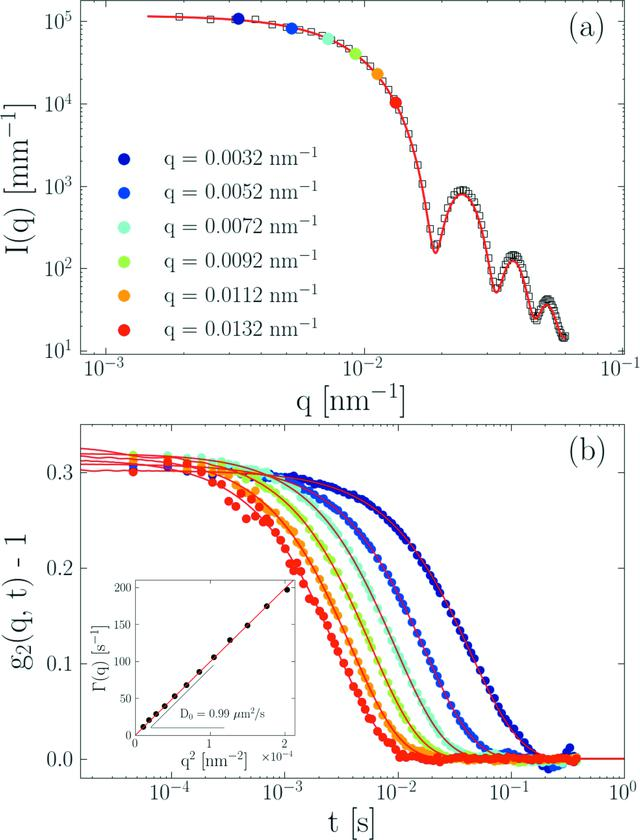disable zoom     view article Figure 3 (a) Azimuthally averaged static scattering intensity, I(q), obtained at a sample-to-detector distance of 30.7 m for silica colloids dispersed in water together with q-values chosen for the determination of the intensity autocorrelation function, g2(q, t), by the PyXPCS programme. The continuous curve corresponds to the polydisperse sphere model with RS = 237 nm and PDI ≃ 1.06. (b) Azimuthally averaged g2(q, t) for the selected q-values measured at 25°C. The color code is the same as that in panel (a). The fitted curves correspond to exponential decay given by equation (3). The inset depicts the q-dependence of the decay rate, Γ(q) = D0q2, with a diffusion coefficient D0 = 0.99 µm2 s−1.JOURNAL OFSYNCHROTRONRADIATION
ISSN: 1600-5775
Volume 25| Part 6| November 2018| Pages 1753-1759